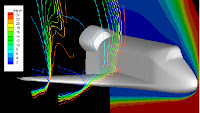### Time Relaxed Monte Carlo (TRMC) simulation of the Boltzmann equationShuttle reentry
Computation of the aerothermodynamics of hypersonic re-entry vehicles along their entire trajectory involves continuum conditions at low altitudes and rarefied, or non-equilibrium, conditions at high altitudes. Accurate 2D and 3D DSMC simulations can require prohibitively high numbers of simulated particles, especially in regions where the Knudsen number is very small.
However, it is precisely in these regions where the collision process can be replaced by a thermodynamic equilibrium approximation. This is the motivation behind developing the Time Relaxed Monte Carlo schemes [1,2] for hypersonic non-equilibrium flows. In the sequel we report some images of the second order TRMC simulations for the flow past an ellipse at Mach number Ma = 20 with Tobj/Tinf = 5 (see reference ). The method allows larger time step computations close to fluid regimes compared to standard DSMC methods. Figures for all simulations using different Knudsen numbers plot the temperature (left) and mean velocity (right). Higher order extension of the present method based on adaptivity and recursivity have been developed in . See also my research activity [here] for further details.

Rarefied regime: Knudsen number=0.1

Intermediate regime: Knudsen number=0.01Fluid limit: Knudsen number=10-6

References
1. L.Pareschi, G.Russo, Time Relaxed Monte Carlo methods for the Boltzmann equation, SIAM J. Sci. Comput. 23 (2001), no 4, 1253--1273
2. L.Pareschi, G.Russo, An introduction to Monte Carlo methods for the Boltzmann equation. ESAIM: Proceedings, Vol.10, pp.35-75 (2001)
3. L.Pareschi, S.Trazzi, Numerical solution of the Boltzmann equation by Time Relaxed Monte Carlo (TRMC) methods, International Journal of Numerical Methods in Fluids, 48, (2005), pp. 947-983.
4. Trazzi, Stefano ; Pareschi, Lorenzo ; Wennberg, Bernt. Adaptive and recursive time relaxed Monte Carlo methods for rarefied gas dynamics. SIAM J. Sci. Comput. 31 (2008/09), no. 2, 1379--1398.